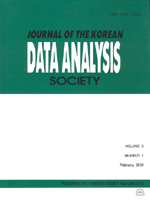상세검색
최근 검색어 전체 삭제
다국어입력
즐겨찾기0KCI등재 학술저널

# Hierarchical Bayesian Model for Small Area Estimation of Poisson Means

• 등재여부 : KCI등재
• 2011.04
• 623 - 631 (9 pages)

Small area estimation is a statistical method to estimate the parameters of subpopulations having small sample sizes. Hierarchical Bayesian approach has been (relatively) recently proposed for small area estimation problems. The Poisson-gamma model is usually used to formulate a dataset consisting of counts. This study investigates the hierarchical Bayes estimators comparing the empirical Bayes estimators of mean rates for the small areas. We explain the suggested method through a practical dataset. We also implement the statistical computations via R2WINBUGS to compare the estimates and their mean squared errors in a Monte Carlo study.

1. Introduction

2. Hierarchical Bayesian Method for Poisson-Gamma Model

3. An Example and Simulation Study

4. Concluding Remarks

References